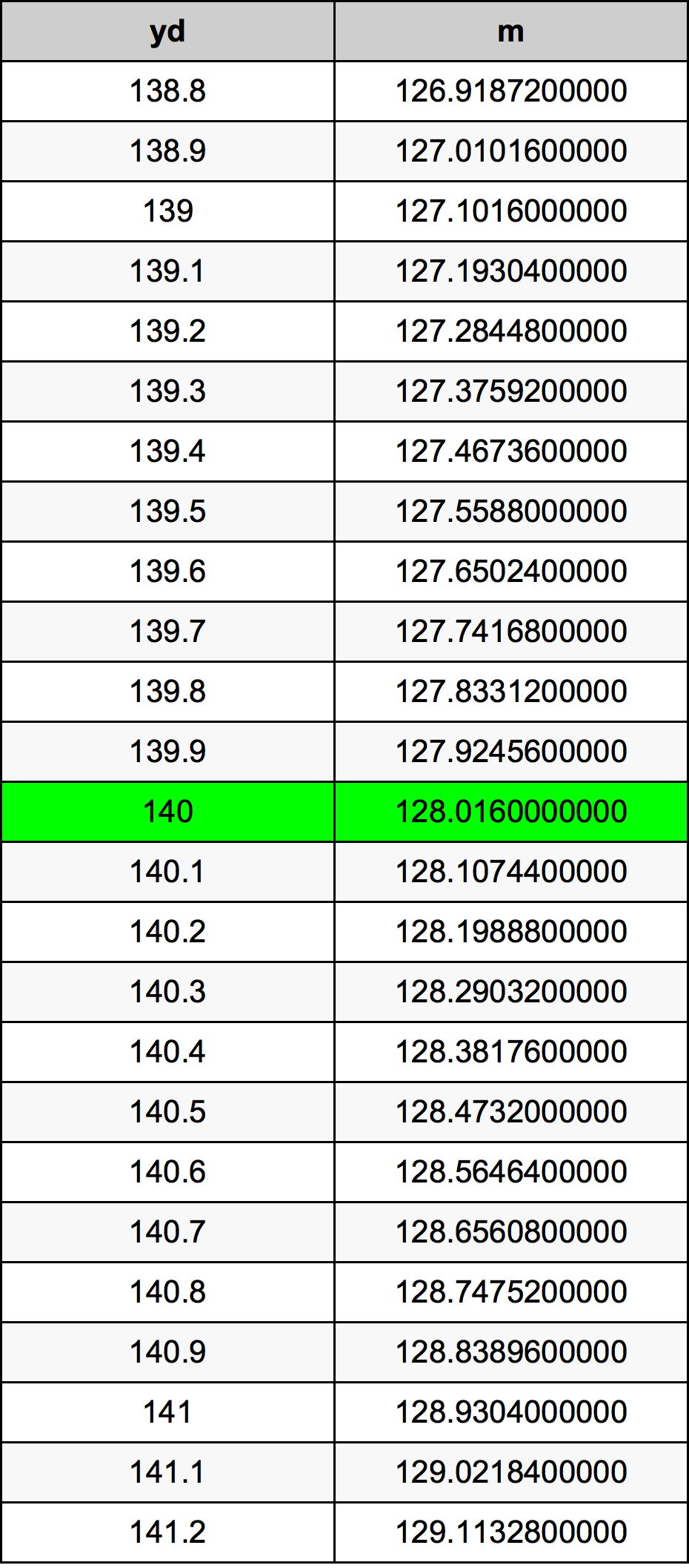Yards To Meters

# 140 yd to m140 Yards to Meters

yd
=
m

## How to convert 140 yards to meters?

 140 yd * 0.9144 m = 128.016 m 1 yd
A common question is How many yard in 140 meter? And the answer is 153.105861767 yd in 140 m. Likewise the question how many meter in 140 yard has the answer of 128.016 m in 140 yd.

## How much are 140 yards in meters?

140 yards equal 128.016 meters (140yd = 128.016m). Converting 140 yd to m is easy. Simply use our calculator above, or apply the formula to change the length 140 yd to m.

## Convert 140 yd to common lengths

UnitUnit of length
Nanometer1.28016e+11 nm
Micrometer128016000.0 µm
Millimeter128016.0 mm
Centimeter12801.6 cm
Inch5040.0 in
Foot420.0 ft
Yard140.0 yd
Meter128.016 m
Kilometer0.128016 km
Mile0.0795454545 mi
Nautical mile0.0691231102 nmi

## What is 140 yards in m?

To convert 140 yd to m multiply the length in yards by 0.9144. The 140 yd in m formula is [m] = 140 * 0.9144. Thus, for 140 yards in meter we get 128.016 m.

## 140 Yard Conversion Table## Alternative spelling

140 yd to m, 140 yd in m, 140 Yards to m, 140 Yards in m, 140 Yard to m, 140 Yard in m, 140 yd to Meter, 140 yd in Meter, 140 Yards to Meters, 140 Yards in Meters, 140 yd to Meters, 140 yd in Meters, 140 Yard to Meter, 140 Yard in Meter